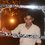# Integration!

Here are some questions, can you please help me solve them?

(A) $\displaystyle \int { \sqrt { \frac { x-3 }{ x-4 } } dx }$

Answer- $\sqrt { (x-3)(x-4) } +\ln { \left( \sqrt { x-3 } +\sqrt { x-4 } \right) } +C$

(B) $\displaystyle \int { \frac { dx }{ { \left[ \left( x-1 \right) \left( 2-x \right) \right] }^{ \frac { 3 }{ 2 } } } }$

Answer- $2\left( \sqrt { \frac { x-1 }{ 2-x } } -\sqrt { \frac { 2-x }{ x-1 } } \right) +C$

(C) $\displaystyle \int { \frac { dx }{ { \left[ { \left( x+2 \right) }^{ 8 }{ \left( x-1 \right) }^{ 6 } \right] }^{ \frac { 1 }{ 7 } } } } dx$

Answer- $\frac { 7 }{ 3 } { \left( \frac { x-1 }{ x+2 } \right) }^{ 1/7 }$

(D) Deduce the reduction formula for ${ I }_{ n }=\displaystyle \int { \frac { dx }{ { \left( 1+{ x }^{ 4 } \right) }^{ n } } }$ and hence evaluate ${ I }_{ 2 }$.

(E) If ${ I }_{ m,n }=\displaystyle \int { \sin ^{ m }{ x } \cos ^{ n }{ x } dx }$ then prove that ${ I }_{ m,n }=\frac { \sin ^{ m+1 }{ x } \cos ^{ n-1 }{ x } }{ m+n } +\frac { n-1 }{ m+n } .{ I }_{ m,n-2 }$

(F) Prove that $\displaystyle \int { \frac { 4{ e }^{ x }+6{ e }^{ -x } }{ 9{ e }^{ x }-4{ e }^{ -x } } } dx=\frac { 35 }{ 36 } \ln { \left| 9{ e }^{ 2x }-4 \right| } -\frac { 3 }{ 2 } x+C$Note by Anandhu Raj
5 years, 2 months ago

This discussion board is a place to discuss our Daily Challenges and the math and science related to those challenges. Explanations are more than just a solution — they should explain the steps and thinking strategies that you used to obtain the solution. Comments should further the discussion of math and science.

When posting on Brilliant:

• Use the emojis to react to an explanation, whether you're congratulating a job well done , or just really confused .
• Ask specific questions about the challenge or the steps in somebody's explanation. Well-posed questions can add a lot to the discussion, but posting "I don't understand!" doesn't help anyone.
• Try to contribute something new to the discussion, whether it is an extension, generalization or other idea related to the challenge.
• Stay on topic — we're all here to learn more about math and science, not to hear about your favorite get-rich-quick scheme or current world events.

MarkdownAppears as
*italics* or _italics_ italics
**bold** or __bold__ bold
- bulleted- list
• bulleted
• list
1. numbered2. list
1. numbered
2. list
Note: you must add a full line of space before and after lists for them to show up correctly
paragraph 1paragraph 2

paragraph 1

paragraph 2

[example link](https://brilliant.org)example link
> This is a quote
This is a quote
    # I indented these lines
# 4 spaces, and now they show
# up as a code block.

print "hello world"
# I indented these lines
# 4 spaces, and now they show
# up as a code block.

print "hello world"
MathAppears as
Remember to wrap math in $$ ... $$ or $ ... $ to ensure proper formatting.
2 \times 3 $2 \times 3$
2^{34} $2^{34}$
a_{i-1} $a_{i-1}$
\frac{2}{3} $\frac{2}{3}$
\sqrt{2} $\sqrt{2}$
\sum_{i=1}^3 $\sum_{i=1}^3$
\sin \theta $\sin \theta$
\boxed{123} $\boxed{123}$

## Comments

Sort by:

Top Newest

For part (A) here is how you can start-

$\displaystyle \int { \sqrt { \frac { x-3 }{ x-4 } } dx }$

Take $\sqrt{x-4}=u,\frac{du}{dx}=\frac{1}{2\sqrt{x-4}}$

The integral becomes,

$\displaystyle 2 \int { \sqrt {u^2+1 } dx }$

Now substitute $u=\tan (m)$,

I think from here you can do it on your own.

- 5 years, 2 months ago

Log in to reply

Yeah! Thank you! :)

- 5 years, 2 months ago

Log in to reply

$\text{Part B :}$

Put $x-1=t^2\implies dx=2t dt$

$I = \int \frac{2t dt}{t^3(1-t^2)^{3/2}}\implies I =2\int \frac{dt}{\sqrt{1-t^2}}\frac{1}{t^2(1-t^2)}$

Put $t=\sin \theta\implies d\theta = \frac{dt}{\sqrt{1-t^2}}\implies I = 2\int \frac{d\theta}{\sin^2 \theta+\cos^2 \theta}\implies I = 2\int \frac{\sin^2 \theta+ \cos^2 \theta}{\sin^2 \theta \cos^2 \theta}d\theta$

$I = 2\int(\sec^2 \theta + \csc^2 \theta) d\theta \implies I = 2(\tan \theta - \cot \theta) + C$

Substitute back $\theta,t$ & get the desired result. Hope it helps

- 5 years, 2 months ago

Log in to reply

×

Problem Loading...

Note Loading...

Set Loading...